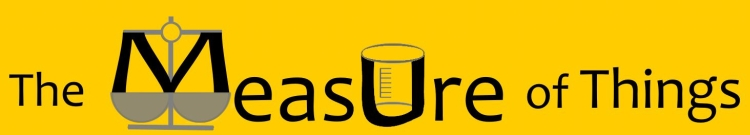Bluebulb Projects presents:Enter a measurement to see comparisons

Equivalents in other units

How heavy is 0.00000600 tons?

Sort Order:
Closest first | Highest first | Lowest first

 It's about as heavy as a Quarter In other words, 0.00000600 tons is 0.96 times the weight of a Quarter, and the weight of a Quarter is 1.04 times that amount. (United States quarter-dollar coin) (a.k.a. Quarter dollar, a.k.a. America the Beautiful quarter, a.k.a. two bits)The America the Beautiful series quarters, which began to enter circulation in 2017, weigh 0.000006250 tons each. In answer to the common riddle, there are 119 ridges around the edge of the coin in its current design. It's about one-and-one-tenth times as heavy as a sheet of Paper In other words, 0.00000600 tons is 1.1 times the weight of a sheet of Paper, and the weight of a sheet of Paper is 0.91 times that amount. (for US Letter, a.k.a. ANSI A; 215.9mm by 279.4mm (8.5 in x 11 in); 20lb)The weight of a ream (500 sheets) of 0.01 tons, 8.5 inch x 11 inch paper is 0.0022 tons, each sheet weighing 0.00000550 tons. Paper of this size has a thickness of 0.004 caliper (0.1 mm). It's about three-fourths as heavy as an Eye (Human) In other words, 0.00000600 tons is 0.77 times the weight of an Eye (Human), and the weight of an Eye (Human) is 1.3 times that amount. (adult) A fully developed eye (in a person over the age of thirteen), weighs about 0.00000780 tons. The pupil of the eye varies depending on the amount of light it is exposed to, but typically measures about 4 mm across. It's about one-and-three-tenths times as heavy as a Die (Dice) In other words, 0.00000600 tons is 1.3 times the weight of a Die (Dice), and the weight of a Die (Dice) is 0.77 times that amount. (for six-sided, 16 mm, rounded cube) A die, of the size most commonly used in board games and casinos, typically weight 0.00000450 tons. The use of dice and dice-like tools predates written history, with the oldest known examples belonging to a 5,000-year old backgammon set uncovered during an archaeological dig in modern-day Iran. It's about two times as heavy as a Penny In other words, 0.00000600 tons is 2.2 times the weight of a Penny, and the weight of a Penny is 0.45 times that amount. (United States) (formally one-cent coin) (Union shield design, 2010-present)The weight of a United States penny is 0.00000280 tons. According to a 2010 report by the United States Mint, the cost of manufacturing and distributing a penny is \$0.0179 — more than its face-value. It's about half as heavy as a Battery (AAA) In other words, 0.00000600 tons is 0.45 times the weight of a Battery (AAA), and the weight of a Battery (AAA) is 2.2 times that amount. (Alkaline)An "AAA" size battery weighs 0.0000130 tons. Batteries are named by the American National Standards Institute (ANSI) Committee C18 and AAA batteries were given their name because they were smaller than the "AA" and "A" size batteries which were invented first. It's about one-third as heavy as a Compact disc In other words, 0.00000600 tons is 0.36 times the weight of a Compact disc, and the weight of a Compact disc is 2.8 times that amount. (a.k.a. CD)A compact disc typically weighs 0.0000170 tons. Data is stored on CDs by encoding into the track a series of tiny pits — each between 3.5 and 850 µm (0.00014 and 0.033 in) in length.